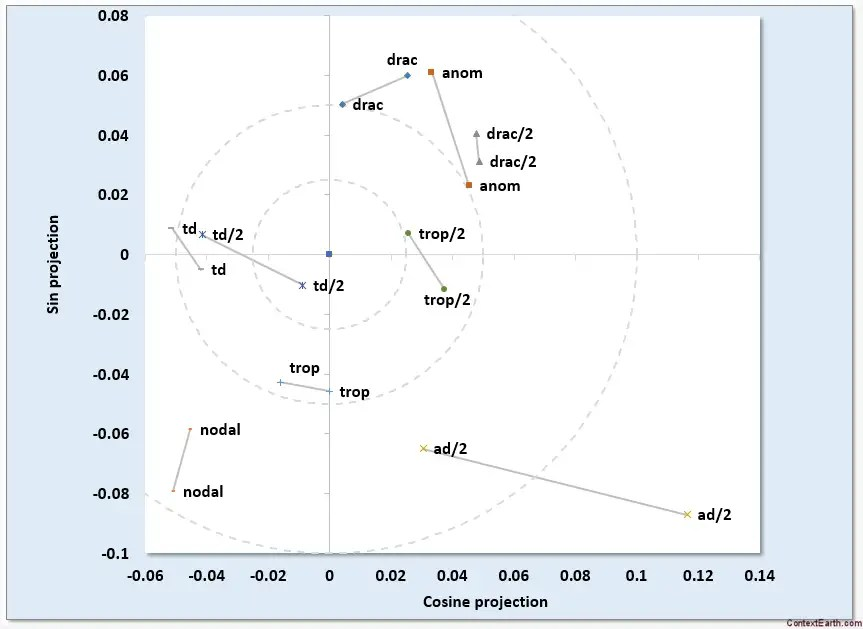# The ENSO Challenge

It’s been quite a challenge decoding the physics of ENSO. Anything that makes the model more complex and with more degrees of freedom needs to be treated carefully. The period doubling bifurcation properties of wave sloshing has been an eye-opener for me. I experimented with adding a sub-harmonic period of 4 years to the 2-year Mathieu modulation and see if that improves the fit. By simply masking the odd behavior around 1981-1983, I came up with this breakdown of the RHS/LHS comparison.This is an iterative solver applied to two completely orthogonal intervals of the ENSO time series leading to largely identical solutions given the fixed tidal factors. The animated gif flips between the fit on one training interval to the orthogonal interval. All that was allowed to change was the amplitude and phase, as shown in the following phasor diagram.Each of the pairs of sinusoidal factors would line up exactly on a phasor diagram if the analyzed process was perfectly stationary. If they line up closely, then there is good agreement — subject to the possibility of overfitting.

So to check for overfitting, we take this same formulation and extend it to regions outside of the ENSO interval from 1880-present. We can’t look to the future, but we can look into the past via the UEP coral proxy records. This is what it looks like.From 1650 to about 1800, the correlation is quite good considering that we only have yearly-averaged values . Over the calibrated proxy interval post 1900 the agreement is as before. However, the 1800’s are out-of-phase (is that due to the amount of volcanic activity during that century Tambora in 1815 plus Krakatoa in 1883?).

This is not conclusive proof but neither does it contradict the model. To achieve such a high correlation between time series separated by at least 200 years is only remotely possible to achieve via random chance.

So is there something fundamental to the 2-year and 4-year period sub-harmonics? I can understand the two year period as being the first doubling of the annual cycle. But the 4 year period would come about from the doubling of the 2-year cycle. This could be recursively applied to 8-year and 16-year periods, but likely not if there was another resonant period close to 4 years.

Is it possible that the 27.55455 day anomalistic lunar tidal period fits into a four year cycle as 13 + 13 + 13 + 13 + 1 = 53 anomalistic tidal periods nearly exactly? 27.55455*53 = 4 * (365.x) where x = 0.098 instead of x=0.242. This is the difference between adding a leap day every fourth year versus adding one every tenth year in terms of alignment. So what the perigee-apogee lunar cycles could do is reinforce this 4-year period by pumping the gravitational cycle in unison with the ocean at the same seasonal reference point.I presented the following chart at the AGU, which showed how machine learning picked out the same aliased anomalistic period from the UEP coral proxy records with no human direction:An angular frequency of 7.821 radians with 12 2π added = 83.219 is close to the 2 π (365.242/27.55455) = 83.285 expected for an anomalistic forcing period.

I also reran a symbolic regression machine learning exercise against 200 years of proxy data from 1650-1790 plus 1915-1975 to see what it would find. No priming of the data was provided except for a biennial modulation with a quad modulation superimposed. This is tricky because the yearly sampling provides a Nyquist limit of two-years — any cycle less than this is indistinguishable from a complementary cycle less than a year.

What the ML did find was a set of multiplied sinusoids at the extreme knee of the Pareto curve. (A multiplied set of sinusoids is simpler in complexity than the additive set because we lose the amplitude on each factor)$cos(130.54192*Year)*cos(118.88917*Year)*cos(102.83697*Year)*cos(-73.662317*Year)$

With the trig identity of multiplying two sinusoids together yielding a pair of +/- periods, this would expand out to 8 cycles. In fact, the values when aliased against the yearly period, yield the following set, shown in the first column:

Learned period Matched against Days
18.21480053 ~ drac-trop long period nodal 18.6 yr 6798
2.928410089 ~ draconic fortnightly 2.907 13.606
1.267545812 ~ drac+trop fortnightly 1.265 13.633
4.234734558 ~ tropical fortnightly 4.235 13.661
2.547488472 ~  1/2 long period nodal or avg(d+t) 2.5479 3399
2.008502387 biennial 2*365.242

A couple are duplicated 18.29 and 1.2679, indicating that those are stronger.

The second column displays the known lunar tidal factors that these are numerically close to when those are also aliased. Notice that they all happen to agree reasonably well with all the known mixed draconic and tropical tidal factors. (See any tidal reference such as by R.D. Ray). This is highly unlikely to occur based on statistical chance unless the lunar cycles have a significant impact — which is exactly what my other non-machine-learning analysis is revealing.

Yet we have to remember that these are only observed if the biennial modulation is applied. That’s the trick to finding the emergent lunar tidal factors in ENSO.

## 2 thoughts on “The ENSO Challenge”

1. Pingback: Tidal Model of ENSO | context/Earth